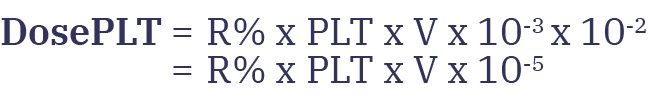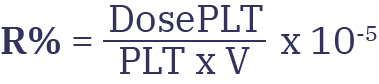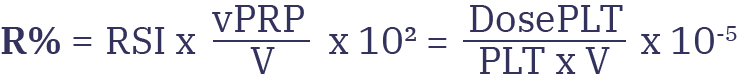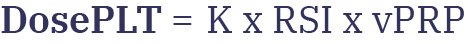# PRP Formula

## A Mathematical Approach

WorldPRP is a Swiss company run by doctors and scientists passionate about PRP. Their intuition dictated that there should be a relationship between the volume of PRP, platelet recovery rate, and useful dose. A few simple mathematical methods enabled the discovery of a general formula still completely unknown to all PRP stakeholders, which will enable all the devices to be qualified precisely and objectively.

The first consideration was that platelet yield is a useful measure provided it can be related to the volume of PRP obtained. Absurdly, a volume of pure blood would get a 100% recovery rate. The second step was to realize that the recovery rate should relate to relative volume, expressed as a ratio to total volume.
This is how the relative strength index (RSI) was invented, with a wink of an eye to the famous eponymous trading index. Thus a volume of whole blood would have an RSI of 100% yield / 100% of volume, or 1. The RSI determines the concentration power of any device. It is therefore very useful to compare effectiveness at a glance.

With R% = platelet yield (in percent) and
vPRP = PRP volume (in ml)
V = volume of blood in the device (in ml)
K = constant

We can therefore write thatHenceConsidering now the Platelet Dose contained in the device (in billions), which is defined by PLT (blood platelet level in G/l) and by the volume V of blood used (in ml). We have:The Useful Dose is defined as:We replace in this formula the term Dose by its value:So we can write:It is elementary to deduce that:By simplifying the expressions we obtain the following general relationship:We can simplify:## Consequences

The relationship is independent of the withdrawal volume, only the PRP volume matters. It's sufficient to know the device's RSI to have an idea of the PLT it can produce. This finally makes it possible to compare the different devices producing PRP in a totally objective way, regardless of the different volumes (sampling or produced PRP).

## A Mathematical Approach

WorldPRP is a Swiss company run by doctors and scientists passionate about PRP. Their intuition dictated that there should be a relationship between the volume of PRP, platelet recovery rate, and useful dose. A few simple mathematical methods enabled the discovery of a general formula still completely unknown to all PRP stakeholders, which will enable all the devices to be qualified precisely and objectively.

The first consideration was that platelet yield is a useful measure provided it can be related to the volume of PRP obtained. Absurdly, a volume of pure blood would get a 100% recovery rate. The second step was to realize that the recovery rate should relate to relative volume, expressed as a ratio to total volume.
This is how the relative strength index (RSI) was invented, with a wink of an eye to the famous eponymous trading index. Thus a volume of whole blood would have an RSI of 100% yield / 100% of volume, or 1. The RSI determines the concentration power of any device. It is therefore very useful to compare effectiveness at a glance.

With R% = platelet yield (in percent) and
vPRP = PRP volume (in ml)
V = volume of blood in the device (in ml)
K = constant

We can therefore write thatHenceConsidering now the Platelet Dose contained in the device (in billions), which is defined by PLT (blood platelet level in G/l) and by the volume V of blood used (in ml). We have:The Useful Dose is defined as:We replace in this formula the term Dose by its value:So we can write:It is elementary to deduce that:By simplifying the expressions we obtain the following general relationship:We can simplify:## Consequences

The relationship is independent of the withdrawal volume, only the PRP volume matters. It's sufficient to know the device's RSI to have an idea of the PLT it can produce. This finally makes it possible to compare the different devices producing PRP in a totally objective way, regardless of the different volumes (sampling or produced PRP).